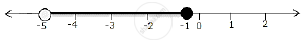Share

Represent the Following In-equalities on Real Number Line : −5 < × ≤ −1 - ICSE Class 10 - Mathematics

ConceptLinear Inequations in One Unknown

Question

Represent the following in-equalities on real number line :

−5 < × ≤ −1

Solution

−5 < × ≤ −1
Solution on number line is :Is there an error in this question or solution?

APPEARS IN

Solution Represent the Following In-equalities on Real Number Line : −5 < × ≤ −1 Concept: Linear Inequations in One Unknown.
S